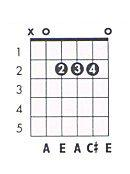# The Chordstore## WHERE THE HEART IS Chords

Transpose up

(INTRO)

F

C

G

F

C

G

(VER)

F
What in the world, what in the world

Are
C
we all doin’ h
G
ere?
F
What do we see, what do we see

When w
C
e look in the mir
G
ror?

(PRE CHO)

‘Cause I
F
’m just lookin’

Lookin’ for s
C
omethin’

t
G
rue

And I believe that
F
I might find it

Maybe I’ll f
C
ind it in yo
G
u

(CHO)

Just show me where the h
F
eart

is
F
(Where the heart is, where the heart is)

Would you show me where the he
G
art

is?
G
(Where the heart is, where the heart is)

I need to feel right where the h
Am
eart

is

(Where the heart is, where the heart is)

Show me where the h
G
eart

is
G
(Where the heart is, where the heart is)

(VER)

Rem
F
ember when you felt the sun?

Remember how the r
C
ain felt down on your sk
G
in?
G
Where’d you lose your sense of wonder?
G

‘Cause there was a
F
time
F
We weren’t thinkin’, we were flyin’

We were al
C
ive

I wanna feel al
G
ive

(PRE CHO)

‘Cause I
F
’m just lookin’

Lookin’ for s
C
omethin’

t
G
rue

And I believe that
F
I might find it

Maybe I’ll
C
find it in yo
G
u

(CHO)

Just show me where the h
F
eart

is
F
(Where the heart is, where the heart is)

Would you show me where the he
G
art

is?
G
(Where the heart is, where the heart is)

I need to feel right where the h
Am
eart

is

(Where the heart is, where the heart is)

Show me where the h
G
eart

is
G
(Where the heart is, where the heart is)

(VER)

F
Show me how to never grow o
D
ld
F
Show me now ‘fore I lose my so
D
ul
F
In a world that’s gone cold
F
In a world that’s gone col
G
d

(BRI)

Show me where the h
F
eart

is

Would you show me where the h
G
eart

is?

I need to feel right where the h
Am
eart

is

Show me where the he
G
art

is

The h
G
eart

is

(CHO)

Just show me where the h
F
eart

is
F
(Where the heart is, where the heart is)

Would you show me where the he
G
art

is?
G
(Where the heart is, where the heart is)

I need to feel right where the h
Am
eart

is

(Where the heart is, where the heart is)

Show me where the h
G
eart

is
G
(Where the heart is, where the heart is)
G
The heart is, yeah

(OUTRO)

(Show me where the he
F
art

is)
F
Show me where the heart is
F
(Show me where the heart is)

I need to feel right where the h
G
eart

is
G
(Show me where the heart is)
G
Show me where the heart is

(Show me where the
Am
heart

is)

T
G
he heart is

Rate this song's chords

?

### Write For Us